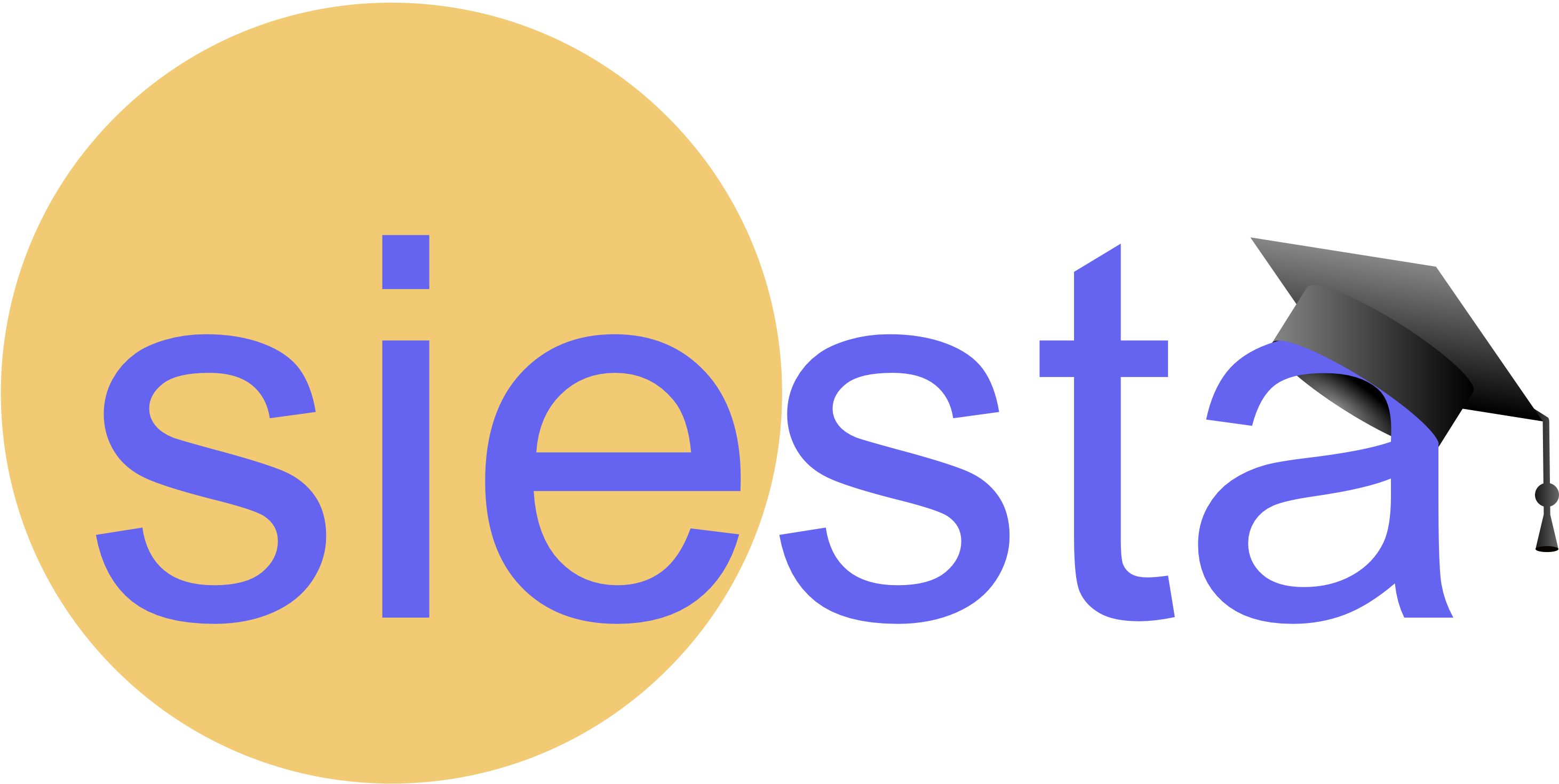The tutorials developed by SIMUNE provide a set of examples introducing Density Functional Theory (DFT) calculations with the SIESTA code. The reader will progressively learn how to perform SIESTA calculations, and how to extract and visualise data from the output. Each tutorial introduces a new feature of DFT calculations emphasizing the scientific value.

Tutorials include scientific background and practical part (pdf), scripts for automated post-processing, examples of input, output files.